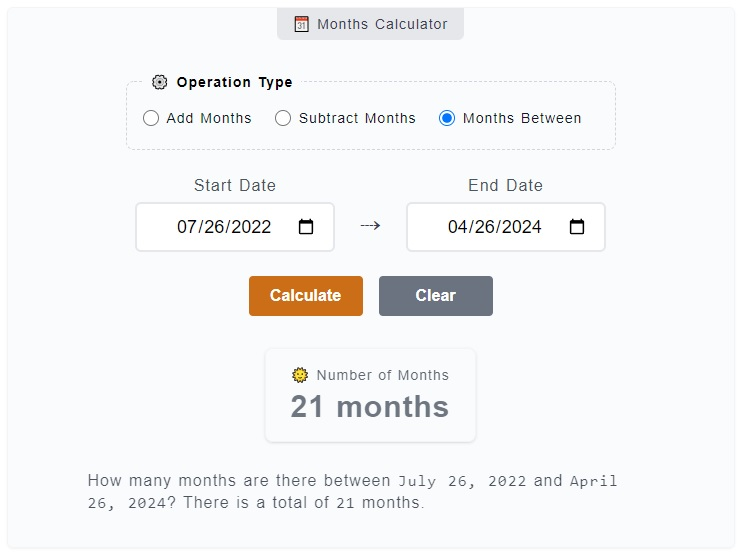# Months Calculator

The Months Calculator has three (3) functionalities, it is used to get the number of months between two dates, add months and subtract months from a reference date. For example, you can add `9 months` or subtract `3` months from the starting date. You can also get the months between and . Just enter these values on the calculator.

🗓️ Months Calculator
⚙️ Operation Type

### 🌞 Number of Months

21 months

How many months are there between `June 23, 2023` and `March 23, 2025`? There is a total of `21` months.

## How to Use the Months Calculator

To use the Months Calculator, you just need to pick first the operation you want to use - add months, subtract months or get the total number of months in between two dates. You just need to enter the values on corresponding inputs and click on `Calculate` button. Here's a more detailed step by step guide.1. Step 1

On the topmost part of the calculator, you can find the `⚙️ Operation Type` field set. It is where you need to choose from the operation options - add months, subtract months and months between. For this example, let's select the `months between` option which is already selected by default.

2. Step 2

After you have chosen an operation type, the next thing to do is to fill the input fields - `Start Date` and `End Date`. The Start Date represents the base or reference date of the count-off while the End Date is where the count-off ends.

3. Step 3

The final step is to click on the `Calculate` button to get your results. You can reset the filled input fields as well by just clicking on the `Clear` button.

In case you are doing some addition or subtraction of months from the starting date, first select the `add` or `subtract` as the type of operation. Then, enter the starting date and the number of days want to add or subtract on their corresponding fields.

Although we have a much specific calculator for months from now (➕ add months) and months ago (➖ subtract months), this calculator has more option to change the starting date and combined with months between two dates operation.